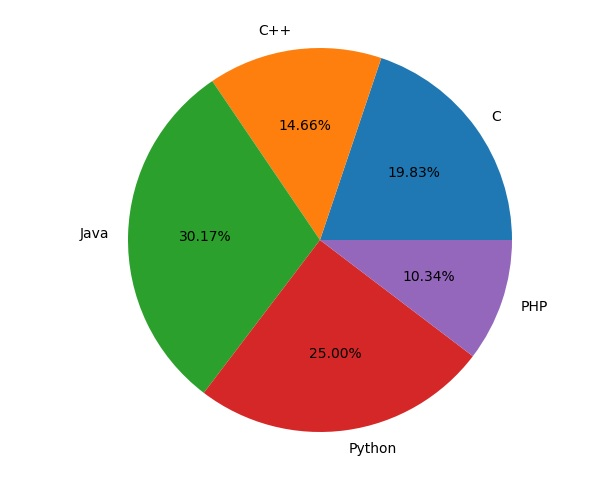# Matplotlib - Pie Chart

A Pie Chart can only display one series of data. Pie charts show the size of items (called wedge) in one data series, proportional to the sum of the items. The data points in a pie chart are shown as a percentage of the whole pie.

Matplotlib API has a pie() function that generates a pie diagram representing data in an array. The fractional area of each wedge is given by x/sum(x). If sum(x)< 1, then the values of x give the fractional area directly and the array will not be normalized. Theresulting pie will have an empty wedge of size 1 - sum(x).

The pie chart looks best if the figure and axes are square, or the Axes aspect is equal.

## Parameters

Following table lists down the parameters foe a pie chart −

 x array-like. The wedge sizes. labels list. A sequence of strings providing the labels for each wedge. Colors A sequence of matplotlibcolorargs through which the pie chart will cycle. If None, will use the colors in the currently active cycle. Autopct string, used to label the wedges with their numeric value. The label will be placed inside the wedge. The format string will be fmt%pct.

Following code uses the pie() function to display the pie chart of the list of students enrolled for various computer language courses. The proportionate percentage is displayed inside the respective wedge with the help of autopct parameter which is set to %1.2f%.

```from matplotlib import pyplot as plt
import numpy as np
fig = plt.figure()